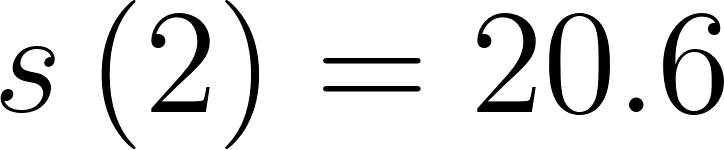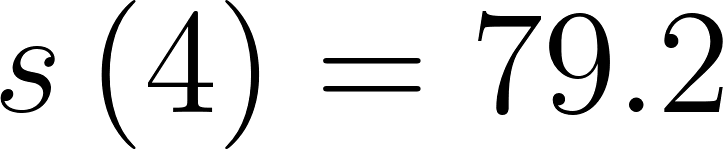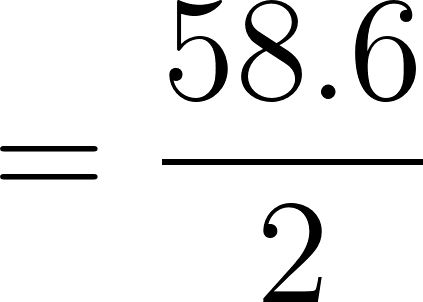×

×

# The table shows the position of a motorcyclist after accelerating from rest. t ssecondsdISBN: 9781305270336 484

## Solution for problem 7 Chapter 2.1

Single Variable Calculus: Early Transcendentals | 8th Edition

• Textbook Solutions
• 2901 Step-by-step solutions solved by professors and subject experts
• Get 24/7 help from StudySoup virtual teaching assistantsSingle Variable Calculus: Early Transcendentals | 8th Edition

4 5 1 344 Reviews
30
5
Problem 7

The table shows the position of a motorcyclist after accelerating from rest. t ssecondsd 0 1 2 3 4 5 6 s (feet) 0 4.9 20.6 46.5 79.2 124.8 176.7 (a) Find the average velocity for each time period: (i) f2, 4g (ii) f3, 4g (iii) f4, 5g (iv) f4, 6g (b) Use the graph of s as a function of t to estimate the instantaneous velocity when t 3.

Step-by-Step Solution:

Problem 7

The table shows the position of a motorcyclist after accelerating from rest.(a) Find the average velocity for each time period: (i) f2, 4g  (ii) f3, 4g   (iii) f4, 5g   (iv) f4, 6g

(b) Use the graph of s as a function of t to estimate the instantaneous velocity when t 3.

Step by Step Solution

Step 1 of 10

Consider the data for the position of a motorcyclist after accelerating from rest.(a)

The objective is to find the average velocity for the time period [2,4],.

(i)

Observe from the table,

At t=2,the positionAt t=4,the positionThe average velocity is ,Therefore the average velocity for the time period [2,4] is 29.3 feet/second.

Step 2 of 10

Step 3 of 10

##### ISBN: 9781305270336

Unlock Textbook Solution